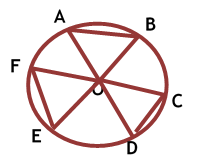Maths / Circles / Equal Chords of a Circle Subtend Equal Angle at the Centre

QUESTION

 In the given figure, if O is the centre of the circle, AOB = EOF = COD = 60^0 and AB = 4 units, find EF + CD.EXPLANATION
Explain TypeExplanation Content
Text
 O is the centre of the circle.AOB = EOF = COD = and AB = 4 units (Given)Here, AOB = EOF = CODTherefore, AB = EF = CD = 4 units [ If the angles subtended by two chords of a circle at the centre are equal then the chords are alos equal]Thus, EF + CD = 4 units + 4 units = 8 units.TestimonialsStudent Feedback on Methodology - Khushal Bindal C/o ABHYAS Academy
6th
The methodology of Abhyas academy is nice. The teachers over here explain us the chapter so nicely and accurately so that we can tell the whole chapter in class before the teacher in the class tell up. They always build us to be the topper.